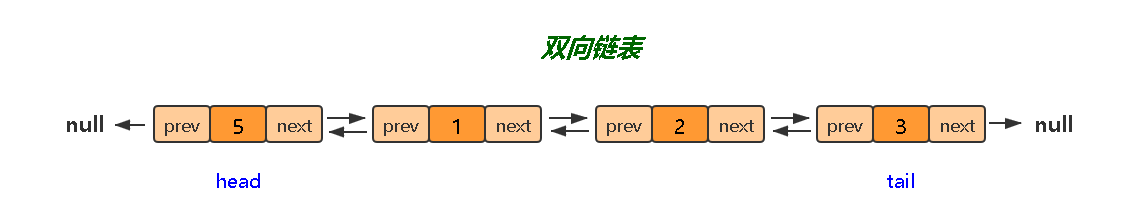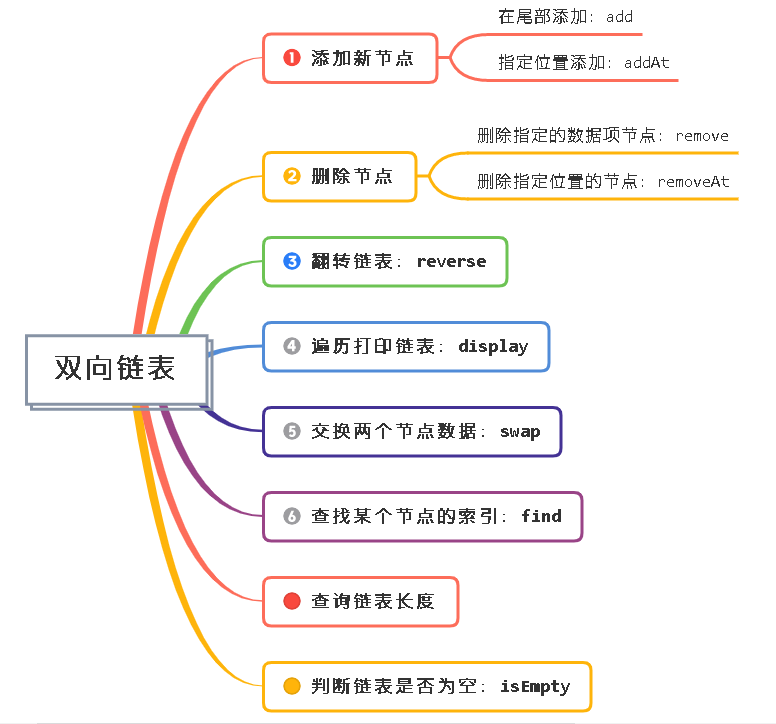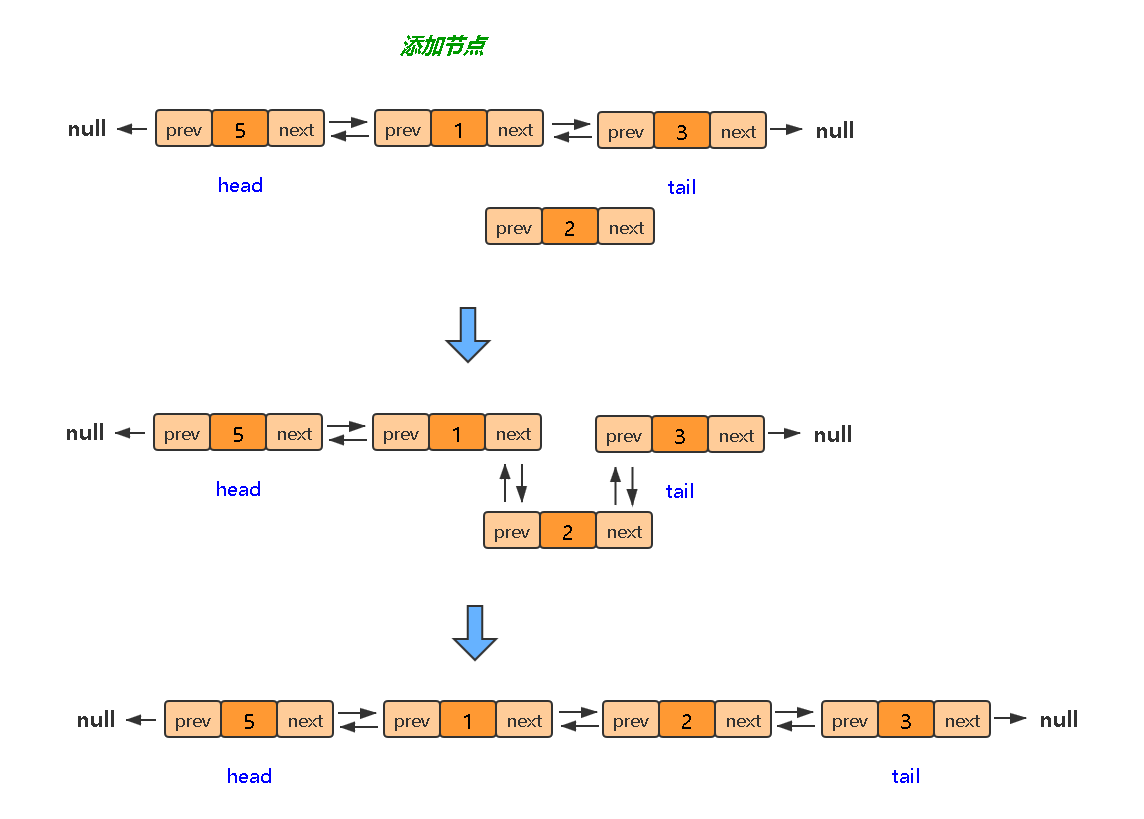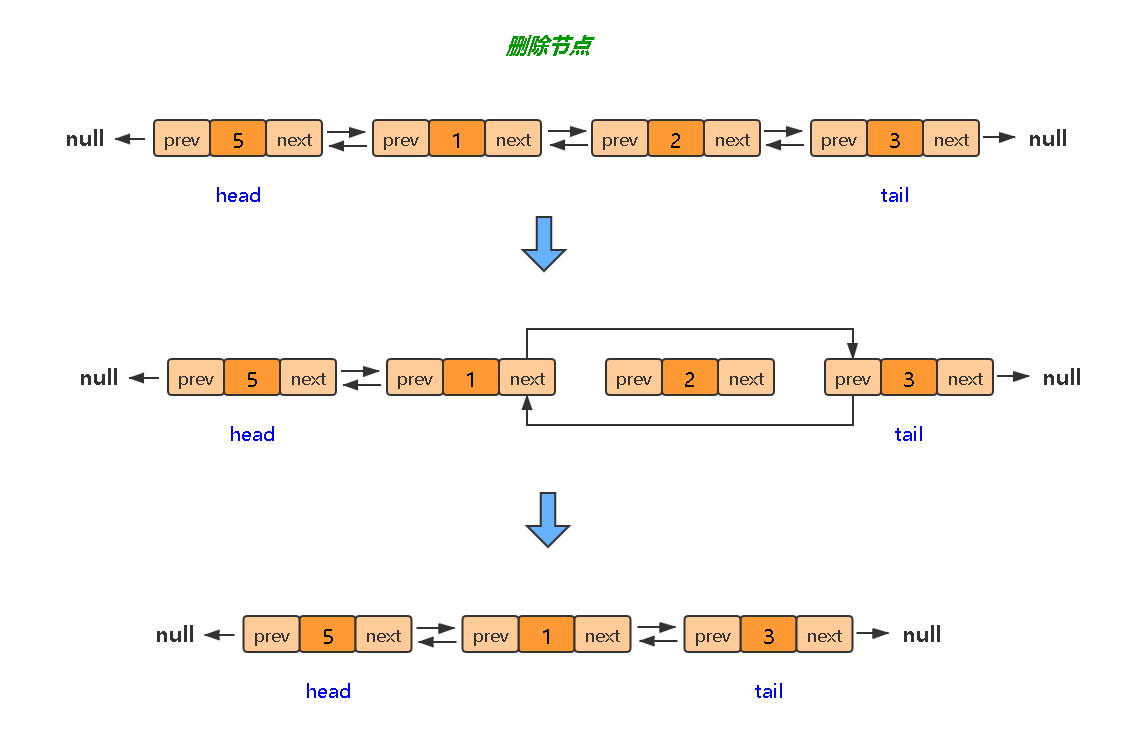## Two way linked list: I'm no longer one-way driving

The journey of ants 2021-05-03 19:20:04
way linked list longer one-way

Unlike a single linked list , Each node of the two-way linked list contains data , It also includes pointers to its front and back nodesIn fact, double linked list is better than single linked list , A pointer to the previous node is added to the node

Now I'll write a double linked list , Implement some methods ：Let's first implement a node constructor ：

``````class Node {
constructor(element) {
this.element = element; // Node data value
this.next = null; // Front pointer
this.prev = null; // The back pointer
}
}
Copy code ``````

## The overall structure of two-way linked list

``````class DoubleLinkedList {
constructor() {
this.count = 0; // Record the number of nodes
this.head = null; // Two way linked list head
this.tail = null; // The tail of a two-way linked list
}
// Add nodes at the end of the list
add(element) {}
// Add a node at the specified location in the linked list
addAt(index, element) {}
// Remove the node at the specified position in the linked list
removeAt(index) {}
// Remove the specified node from the list
remove(element) {}
// Flip list
reverse() {}
// Swap the positions of the two nodes
swap() {}
// Traversing the linked list
traverse() {}
// Find the index of a node
find() {}
// Check whether the linked list is empty
isEmpty() {}
// The length of the query list
length() {}
}
Copy code ``````

The next step is to realize one by one

According to the location （ Indexes ） Find node , The next method will always use this method , Just encapsulate it as a method , In order to call

``````getElement(index) {
if (index >= 0 && index < this.count) {
let node = this.head;
// Loop to the node
for (let i = 0; i < index; i++) {
node = node.next;
}
return node;
}
return undefined;
}
Copy code ``````

## 1、 Add a node### 1.1 Add a node at the tail

``````// Pass in a node
add(element) {
// Create a node first
const node = new Node(element);
// If the list is empty , That is, both the head node and the tail node are node 了
if (this.head === null && this.tail === null) {
this.head = node;
this.tail = node
}
// If the linked list already has a head , Then add a node at the tail
else {
node.prev = this.tail;
this.tail.next = node;
this.tail = node;
}
// Total number of nodes +1
this.count++;
}
Copy code ``````

### 1.2 Add a node at the specified location in the linked list

``````addAt(index, element) {
if (index >= 0 && index <= this.count) {
const node = new Node(element);
// When inserted in the head
if (index === 0) {
this.head.prev = node;
node.next = this.head;
this.head = node;
}
// Insert nodes at the end
else if (index === this.count) {
this.add(element)
this.count--;
}
// Insert nodes in the middle
else {
let current = this.getElement(index);
// First, set the relationship between the new node and the previous node
node.prev = current.prev;
current.prev.next = node;
// Then set the new relationship with the next node
node.next = current;
current.prev = node
}
this.count++;
return true
}
return false;
}
Copy code ``````

## 2、 Remove node### 2.1、 Remove the node at the specified position in the linked list

``````removeAt(index) {
if (index >= 0 && index < this.count) {
// Remove the head node
if (index === 0) {
this.head = this.head.next;
this.head.prev = null;
}
// Remove tail nodes
else if (index === this.count - 1) {
this.tail = this.tail.prev;
this.tail.next = null;
}
// Remove intermediate nodes
else {
let current = this.getElement(index);
current.next.prev = current.prev
current.prev.next = current.next
}
this.count--;
return true
}
return undefined;
}
Copy code ``````

### 2.2、 Remove the specified node from the list

Remove the specified node , The first step is to find the node

``````remove(element) {
let current = this.head;
while (current) {
if (current === element) {
// A linked list has only one node
if (current === this.head && current === this.prev) {
this.head = null;
this.tail = null;
}
// Remove the head node
else if (current === this.head) {
this.head = this.head.next;
this.head.prev = null;
}
// Remove tail nodes
else if (current === this.tail) {
this.tail = this.tail.prev;
this.tail.next = null;
}
// Remove intermediate nodes
else {
current.next.prev = current.prev;
current.prev.next = current.next;
}
this.count--;
}
current = current.next;
}
}
Copy code ``````

## 5、 Flip list

``````reverse() {
let current = this.head;
let prev = null;
// First put the middle
while (current) {
let next = current.next;
// hysteron proteron
current.next = prev;
current.prev = next;
prev = current;
current = next
}
this.tail = this.head;
this.head = prev
}
Copy code ``````

## 6、 Swap the positions of the two nodes

``````swap(index1, index2) {
if (index1 >= 0 && index1 < this.count && index2 >= 0 && index2 < this.count) {
let node1 = this.getElement(index1)
let node2 = this.getElement(index2)
// Give Way index1 Always less than index2, Convenient for later search and exchange
if (index1 > index2) {
return this.swap(index2, index1)
}
// Exchange the values of two nodes
[node1.element, node2.element] = [node2.element, node1.element]
return true
}
return undefined;
}
Copy code ``````

## 7、 Traversing the linked list

``````display() {
if (!this.isEmpty()) {
let current = this.head;
let result = '';
while (current) {
result += current.element
current = current.next
if (current) {
result += '->';
}
}
return result;
}
return undefined;
}
Copy code ``````

## 8、 Find the index of a node

``````find(element) {
let current = this.head;
let index = 0
while (current) {
if (current === element) {
return index;
}
current = current.next;
index++;
}
return undefined
}
Copy code ``````

## 9、 Check whether the linked list is empty

``````isEmpty() {
return this.count === 0
}
Copy code ``````

## 10、 The length of the query list

``````length() {
return this.count;
}
Copy code ``````

Integration ：

``````// Implement a node constructor
class Node {
constructor(element) {
this.element = element;
this.next = null;
this.prev = null;
}
}
// Double linked list
class DoubleLinkedList {
constructor() {
this.count = 0; // Record the number of nodes
this.head = null; // Two way linked list head
this.tail = null; // The tail of a two-way linked list
}
// Traverse a method , Loop to the target location
getElement(index) {
if (index >= 0 && index < this.count) {
let node = this.head;
for (let i = 0; i < index; i++) {
node = node.next;
}
return node;
}
return undefined;
}
// Add nodes at the end of the list
add(element) {
// Create a node first
const node = new Node(element);
// If the list is empty
if (this.head === null && this.tail === null) {
this.head = node;
this.tail = node
}
// If the linked list already has a head , Then add a node at the tail
else {
node.prev = this.tail;
this.tail.next = node;
this.tail = node;
}
this.count++;
}
// Add a node at the specified location in the linked list
addAt(index, element) {
if (index >= 0 && index <= this.count) {
const node = new Node(element);
// When inserted in the head
if (index === 0) {
this.head.prev = node;
node.next = this.head;
this.head = node;
} else if (index === this.count) {
this.add(element)
this.count--;
}
// When the head is not inserted
else {
let current = this.getElement(index);
// First, set the relationship between the new node and the previous node
node.prev = current.prev;
current.prev.next = node;
// Then set the new relationship with the next node
node.next = current;
current.prev = node
}
this.count++;
return true
}
return false;
}
// Remove the node at the specified position in the linked list
removeAt(index) {
if (index >= 0 && index < this.count) {
// Remove the head node
if (index === 0) {
this.head = this.head.next;
this.head.prev = null;
}
// Remove tail nodes
else if (index === this.count - 1) {
this.tail = this.tail.prev;
this.tail.next = null;
}
// Remove intermediate nodes
else {
let current = this.getElement(index);
current.next.prev = current.prev
current.prev.next = current.next
}
this.count--;
return true
}
return undefined;
}
// Remove the specified node from the list
remove(element) {
let current = this.head;
while (current) {
if (current === element) {
// A linked list has only one node
if (current === this.head && current === this.prev) {
this.head = null;
this.tail = null;
}
// Remove the head node
else if (current === this.head) {
this.head = this.head.next;
this.head.prev = null;
}
// Remove tail nodes
else if (current === this.tail) {
this.tail = this.tail.prev;
this.tail.next = null;
}
// Remove intermediate nodes
else {
current.next.prev = current.prev;
current.prev.next = current.next;
}
this.count--;
}
current = current.next;
}
}
// Flip list
reverse() {
let current = this.head;
let prev = null;
while (current) {
let next = current.next;
// hysteron proteron
current.next = prev;
current.prev = next;
prev = current;
current = next
}
this.tail = this.head;
this.head = prev
}
// Swap the positions of the two nodes
swap(index1, index2) {
if (index1 >= 0 && index1 < this.count && index2 >= 0 && index2 < this.count) {
let node1 = this.getElement(index1)
let node2 = this.getElement(index2)
// Give Way index1 Always less than index2, Convenient for later search and exchange
if (index1 > index2) {
return this.swap(index2, index1)
}
// Exchange the values of two nodes
[node1.element, node2.element] = [node2.element, node1.element]
return true
}
return undefined;
}
// Traversing the linked list
display() {
if (!this.isEmpty()) {
let current = this.head;
let result = '';
while (current) {
result += current.element
current = current.next
if (current) {
result += '->';
}
}
return result;
}
return undefined;
}
// Find the index of a node
find(element) {
let current = this.head;
let index = 0
while (current) {
if (current === element) {
return index;
}
current = current.next;
index++;
}
return undefined
}
// Check whether the linked list is empty
isEmpty() {
return this.count === 0
}
// The length of the query list
length() {
return this.count;
}
}
Copy code ``````

Let's call ：

``````// First instantiate a bidirectional linked list object
let DLL = new DoubleLinkedList();
// Tail add
DLL.add(1);
DLL.add(2);
// Add... Anywhere
DLL.addAt(0, 3);
DLL.addAt(3, 6);
// Traverse
console.log(DLL.display()); // 3->1->2->6
// Delete... According to location
DLL.removeAt(3);
// Swap two nodes
DLL.swap(0, 2)
console.log(DLL.display()); // 2->1->3
// Flip
DLL.reverse();
// Is the list empty
console.log(DLL.isEmpty()); // false
// Chain length
console.log(DLL.length()); // 3
Copy code ``````

summary ：

• So you'll find that implementing a linked list is nothing more than adding （ Add a node ） Delete （ Delete node ） Change （ In exchange for ） check （ Traverse ）
• Adding and deleting nodes is nothing more than modifying the pointer of nodes

https://qdmana.com/2021/05/20210503191542840o.html